# Fractions Order fractions fractions of amounts Objectives Day

• Slides: 35Fractions Order fractions; fractions of amounts Objectives Day 1 Find equivalent fractions; simplify fractions using multiples and factors Day 2 Compare fractions with related denominators Compare and order fractions with unrelated denominators. Day 3 Find unit and non-unit fractions of amounts. © hamilton-trust. org. uk 1 Year 5/6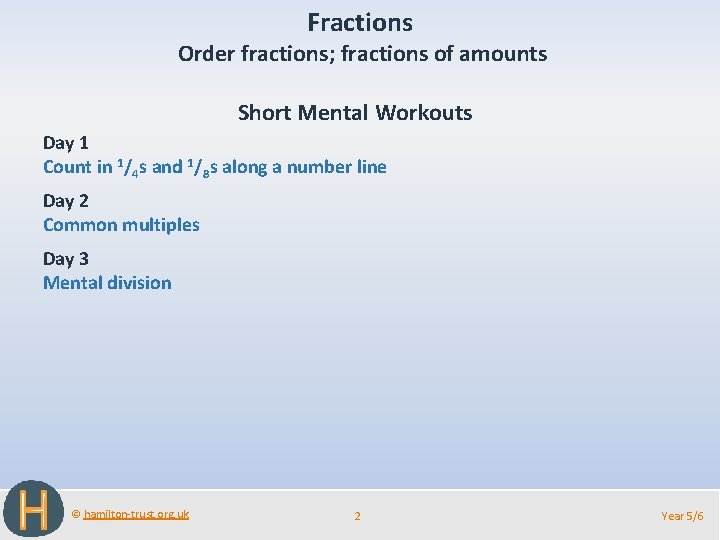Fractions Order fractions; fractions of amounts Short Mental Workouts Day 1 Count in 1/4 s and 1/8 s along a number line Day 2 Common multiples Day 3 Mental division © hamilton-trust. org. uk 2 Year 5/6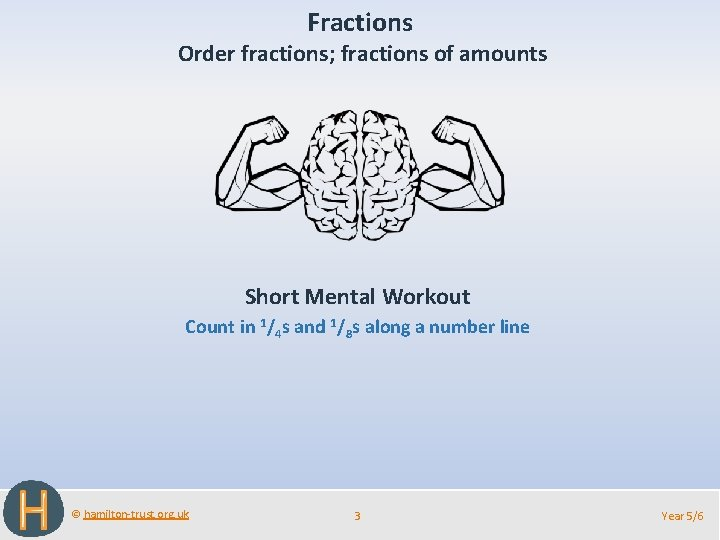Fractions Order fractions; fractions of amounts Short Mental Workout Count in 1/4 s and 1/8 s along a number line © hamilton-trust. org. uk 3 Year 5/6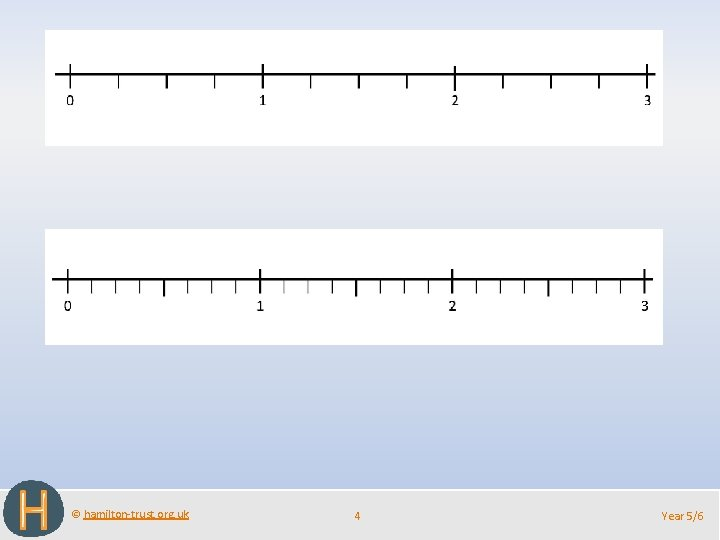© hamilton-trust. org. uk 4 Year 5/6Fractions Order fractions; fractions of amounts Short Mental Workout Common multiples © hamilton-trust. org. uk 5 Year 5/6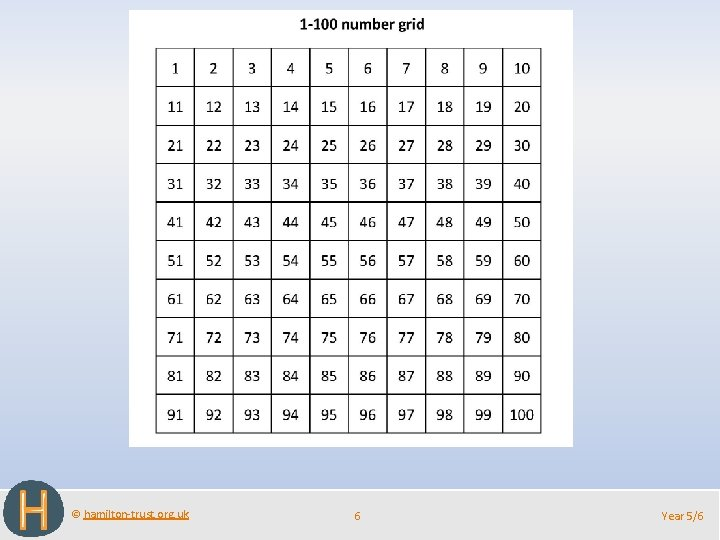© hamilton-trust. org. uk 6 Year 5/6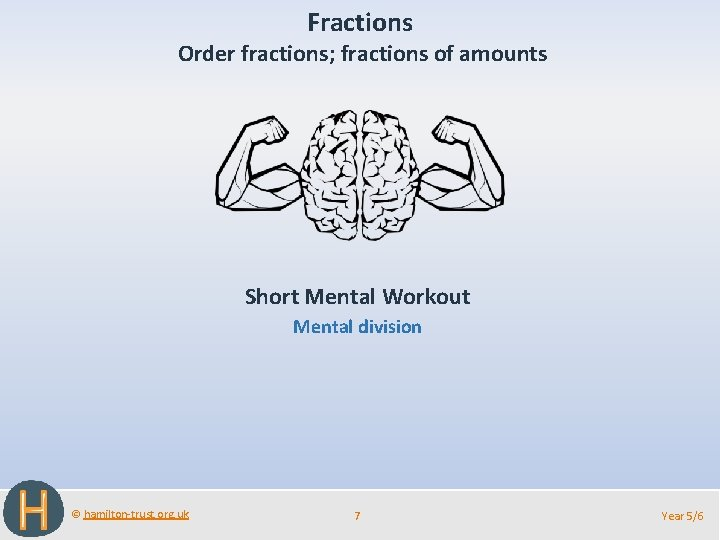Fractions Order fractions; fractions of amounts Short Mental Workout Mental division © hamilton-trust. org. uk 7 Year 5/6Fractions Order fractions; fractions of amounts Objectives Day 1 Find equivalent fractions; simplify fractions using multiples and factors © hamilton-trust. org. uk 8 Year 5/6Day 1: Find equivalent fractions; simplify fractions using multiples and factors; Find equivalent fractions; simplify fractions using multiples and factors Work with a partner to find as many fractions equivalent to 1/ as you can on this fraction 4 wall. Write them on a whiteboard. CHALLENGE! Work in pairs to write fractions equivalent to ¹/4 which are NOT on the fraction wall… What do you notice about your list of fractions? If we multiply both the numerator and denominator by the same number we’ll get an equivalent fraction! © hamilton-trust. org. uk 9 Year 5/6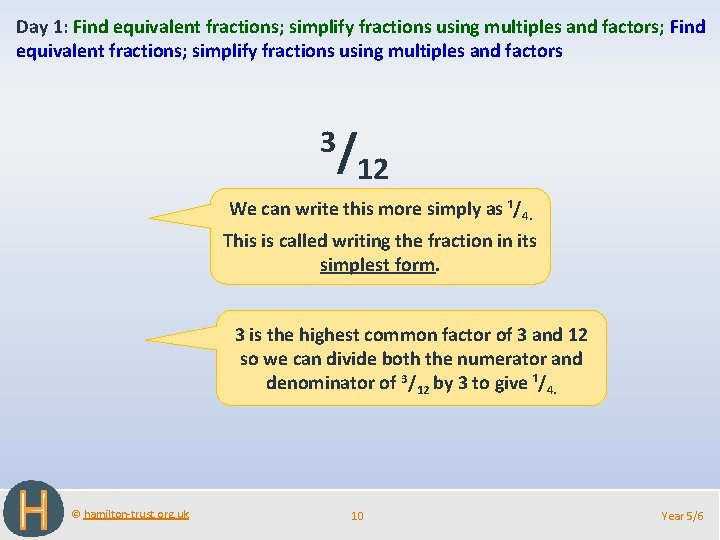Day 1: Find equivalent fractions; simplify fractions using multiples and factors; Find equivalent fractions; simplify fractions using multiples and factors 3/ 12 We can write this more simply as ¹/4. This is called writing the fraction in its simplest form. 3 is the highest common factor of 3 and 12 so we can divide both the numerator and denominator of 3/12 by 3 to give ¹/4. © hamilton-trust. org. uk 10 Year 5/6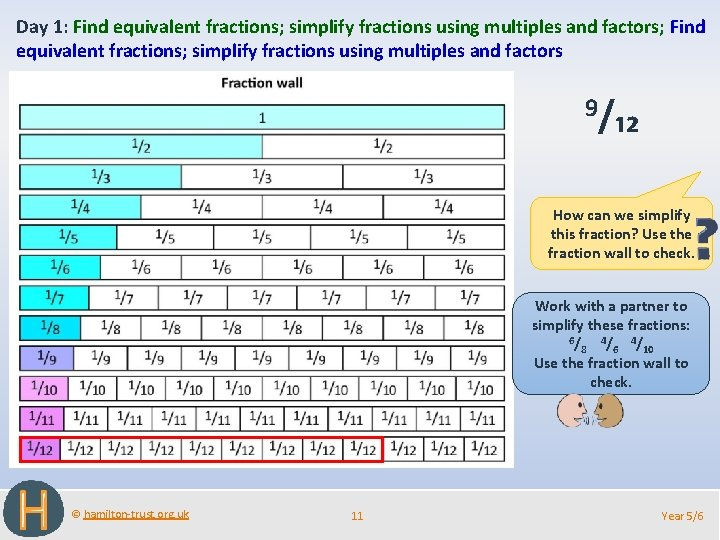Day 1: Find equivalent fractions; simplify fractions using multiples and factors; Find equivalent fractions; simplify fractions using multiples and factors 9/₁₂ How can we simplify this fraction? Use the fraction wall to check. Work with a partner to simplify these fractions: 6/ 4/ 8 6 10 Use the fraction wall to check. © hamilton-trust. org. uk 11 Year 5/6Challenge © hamilton-trust. org. uk 12 Year 5/6© hamilton-trust. org. uk 13 Year 5/6Challenge © hamilton-trust. org. uk 14 Year 5/6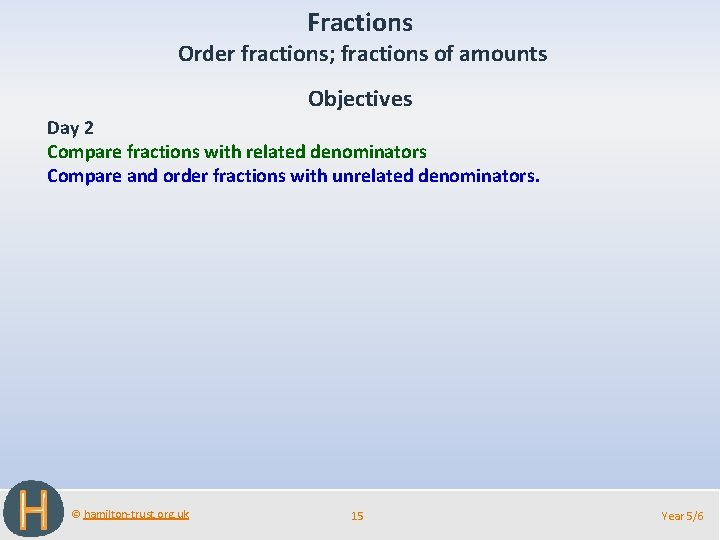Fractions Order fractions; fractions of amounts Objectives Day 2 Compare fractions with related denominators Compare and order fractions with unrelated denominators. © hamilton-trust. org. uk 15 Year 5/6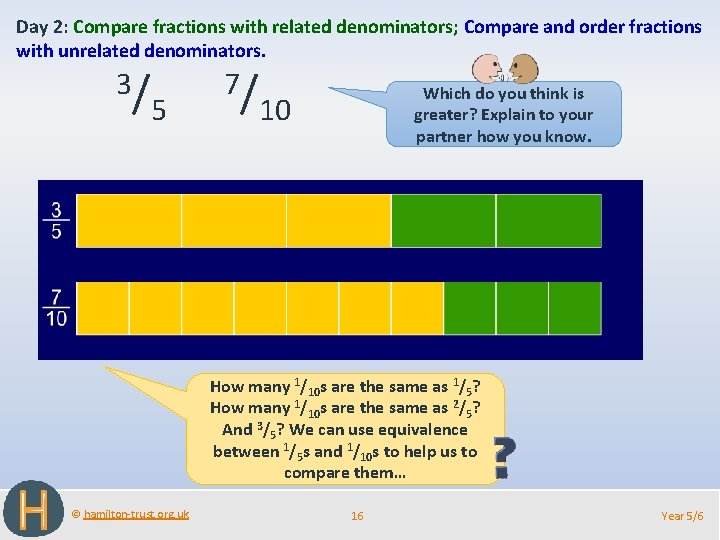Day 2: Compare fractions with related denominators; Compare and order fractions with unrelated denominators. 3/ 5 7/ Which do you think is greater? Explain to your partner how you know. 10 How many 1/10 s are the same as 1/5? How many 1/10 s are the same as 2/5? And 3/5? We can use equivalence between 1/5 s and 1/10 s to help us to compare them… © hamilton-trust. org. uk 16 Year 5/6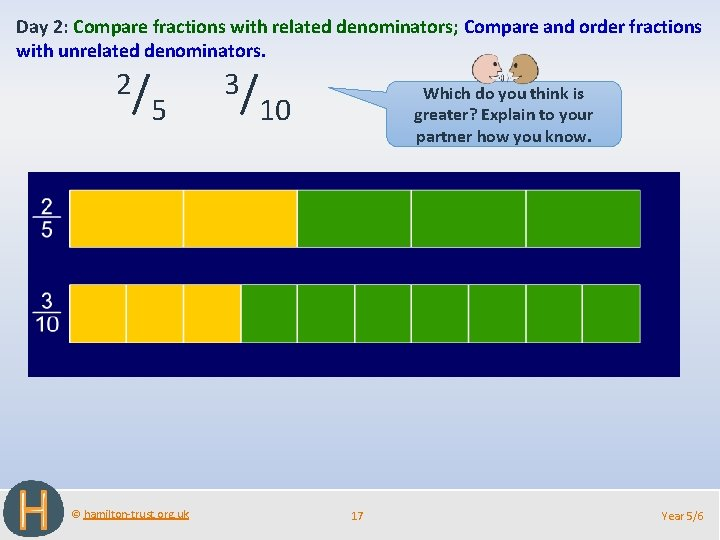Day 2: Compare fractions with related denominators; Compare and order fractions with unrelated denominators. 2/ 5 © hamilton-trust. org. uk 3/ Which do you think is greater? Explain to your partner how you know. 10 17 Year 5/6Day 2: Compare fractions with related denominators; Compare and order fractions with unrelated denominators. See how 1/6 is half the size of 1/3. How many 1/6 s are the same as 2/3? Which is greater 5/6 or 2/3? © hamilton-trust. org. uk 18 Year 5/6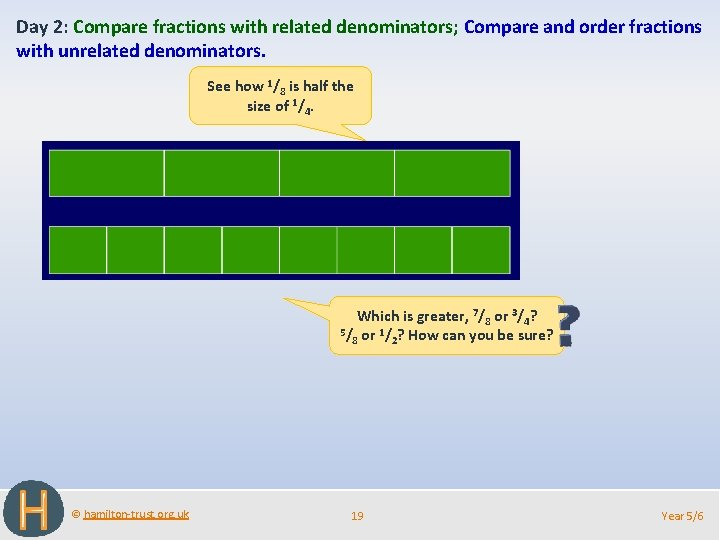Day 2: Compare fractions with related denominators; Compare and order fractions with unrelated denominators. See how 1/8 is half the size of 1/4. Which is greater, 7/8 or 3/4? 5/ or 1/ ? How can you be sure? 8 2 © hamilton-trust. org. uk 19 Year 5/6Challenge © hamilton-trust. org. uk 20 Year 5/6Day 2: Compare and order fractions with unrelated denominators. 2/ 3 3/ Which fraction is bigger? Try to convince your partner. 4 We can’t write 1/3 s as 1/4 s or vice versa BUT we can write both 2/3 and 3/4 as the same sort of fraction. What sort of fraction might that be? HINT! 3 and 4 are both factors of… You could divide a strip into 1/3 s and the same length strip into 1/4 s and show 2/3 is less than 3/4. Now we can write both fractions as 1/12 s. 12! 8/ 12 < 9/ 12 We proved it! 2/ < 3/. 3 4 = © hamilton-trust. org. uk 21 = Year 5/6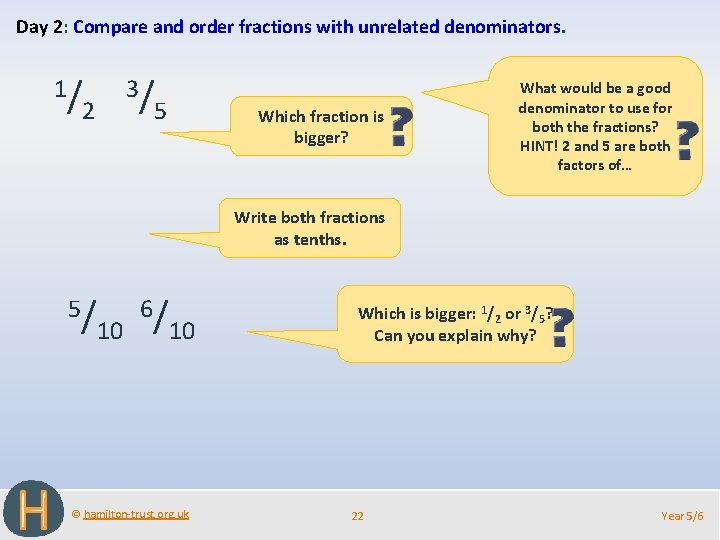Day 2: Compare and order fractions with unrelated denominators. 1/ 3/ 2 5 Which fraction is bigger? What would be a good denominator to use for both the fractions? HINT! 2 and 5 are both factors of… Write both fractions as tenths. 5/ 10 6/ 10 © hamilton-trust. org. uk Which is bigger: 1/2 or 3/5? Can you explain why? 22 Year 5/6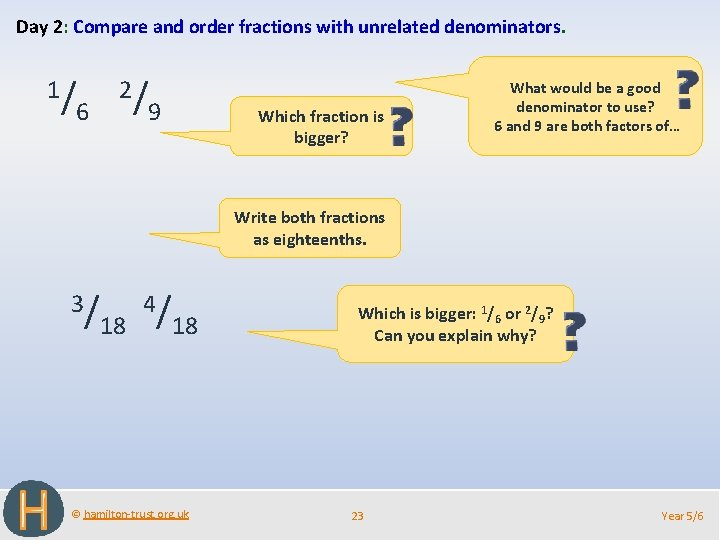Day 2: Compare and order fractions with unrelated denominators. 1/ 6 2/ 9 Which fraction is bigger? What would be a good denominator to use? 6 and 9 are both factors of… Write both fractions as eighteenths. 3/ 18 4/ 18 © hamilton-trust. org. uk Which is bigger: 1/6 or 2/9? Can you explain why? 23 Year 5/6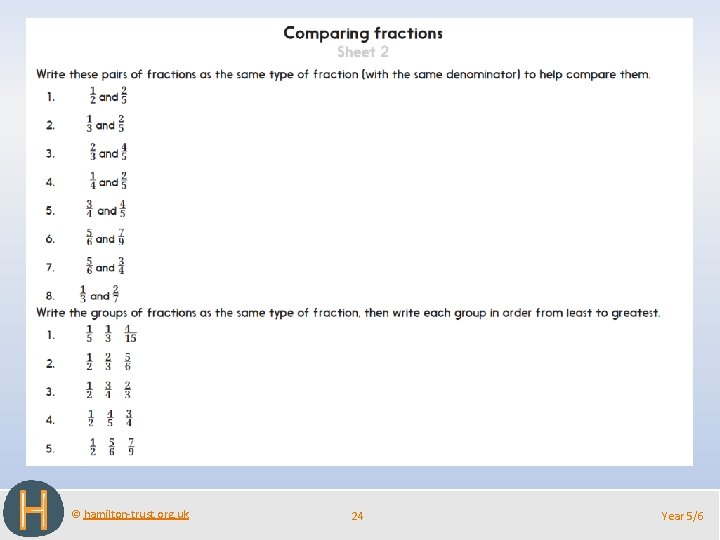© hamilton-trust. org. uk 24 Year 5/6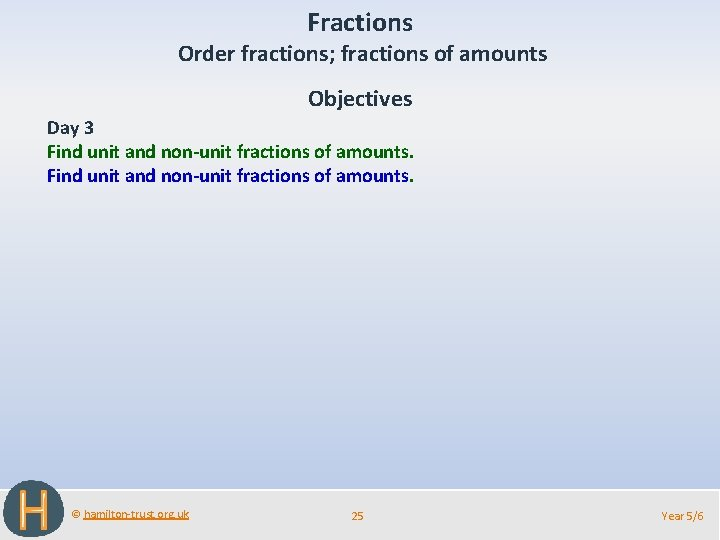Fractions Order fractions; fractions of amounts Objectives Day 3 Find unit and non-unit fractions of amounts. © hamilton-trust. org. uk 25 Year 5/6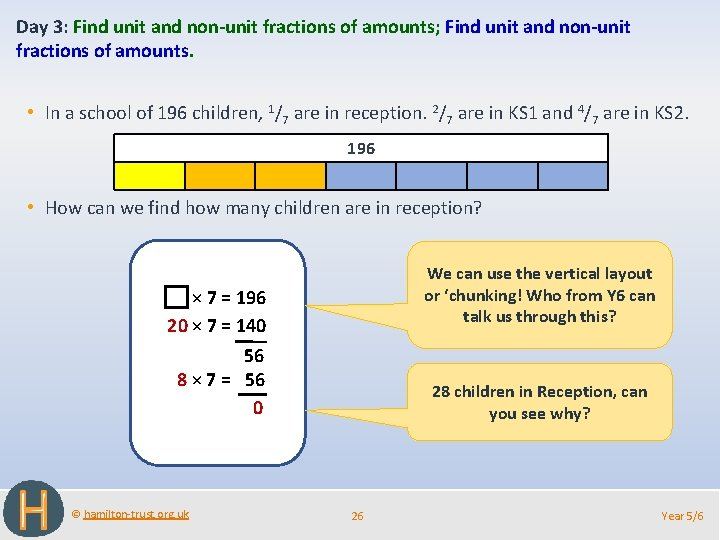Day 3: Find unit and non-unit fractions of amounts; Find unit and non-unit fractions of amounts. • In a school of 196 children, 1/7 are in reception. 2/7 are in KS 1 and 4/7 are in KS 2. 196 • How can we find how many children are in reception? We can use the vertical layout or ‘chunking! Who from Y 6 can talk us through this? × 7 = 196 20 × 7 = 140 56 8 × 7 = 56 0 © hamilton-trust. org. uk 28 children in Reception, can you see why? 26 Year 5/6Day 3: Find unit and non-unit fractions of amounts; Find unit and non-unit fractions of amounts. • In a school of 196 children, 1/7 are in reception. 2/7 are in KS 1 and 4/7 are in KS 2. 196 28 28 • How can we find how many children are in KS 1? Work with a partner to find how many children are in KS 2. © hamilton-trust. org. uk 27 Year 5/6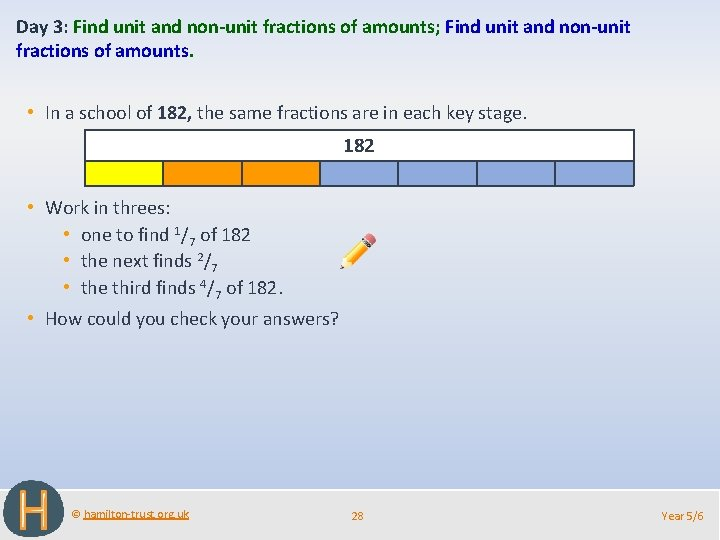Day 3: Find unit and non-unit fractions of amounts; Find unit and non-unit fractions of amounts. • In a school of 182, the same fractions are in each key stage. 182 • Work in threes: • one to find 1/7 of 182 • the next finds 2/7 • the third finds 4/7 of 182. • How could you check your answers? © hamilton-trust. org. uk 28 Year 5/6© hamilton-trust. org. uk 29 Year 5/6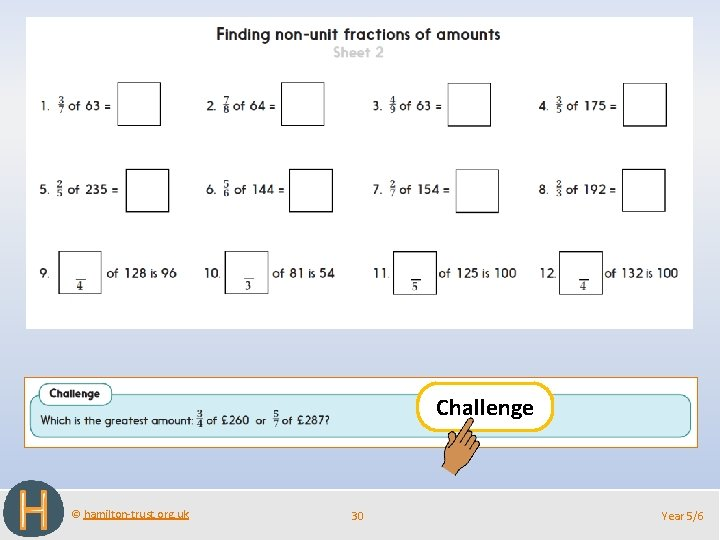Challenge © hamilton-trust. org. uk 30 Year 5/6Fractions Order fractions; fractions of amounts Well Done! You’ve completed this unit. Objectives Day 1 Find equivalent fractions; simplify fractions using multiples and factors Day 2 Compare fractions with related denominators Compare and order fractions with unrelated denominators. Day 3 Find unit and non-unit fractions of amounts. © hamilton-trust. org. uk 31 Year 5/6Problem solving and reasoning questions Year 5 Write three fractions equivalent to 20/25. Describe any pattern in the denominators. Write the missing numbers to make each sentence true. ☐/ 4/ 6 = ☐ < ☐/ 5/ 6 > ☐ ☐ 7/ 10 Find 3/4 of 148: 148 Find 5/8 of 256: © hamilton-trust. org. uk 256 32 Year 5/6Problem solving and reasoning answers Year 5 Write three fractions equivalent to 20/25. 40/50 4/5 8/10 12/15 etc. Describe any pattern in the denominators. The denominators are all multiples of 5. Write the missing numbers to make each sentence true. 2/ 6 = 8/ 4/ ☐ < 5/ 6 > 24 5/ 7/ ☐ Various answers possible, e. g. 4/9 < 5/6 10 Find 3/4 of 148: 1/4 of 148 = 37; 3/4 of 148 = 3 x 37 = 111 37 148 37 37 37 Find 5/8 of 256: 1/8 of 256 = 32; 5/8 of 256 = 5 x 32 = 160 32 © hamilton-trust. org. uk 32 32 256 33 32 32 32 Year 5/6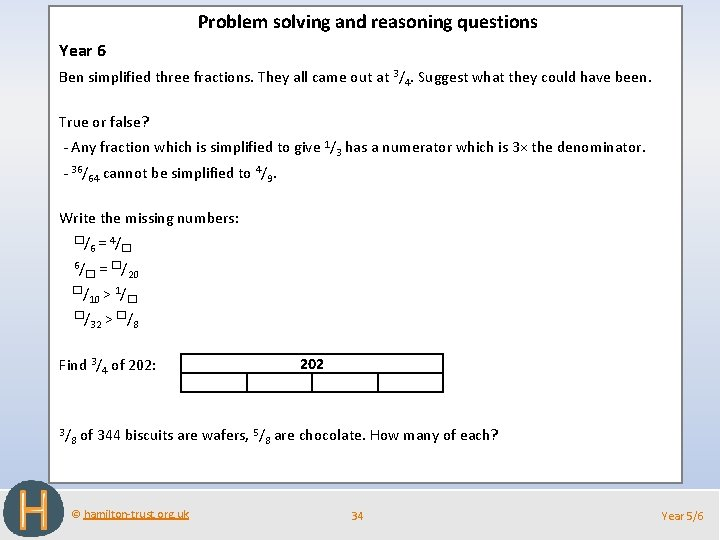Problem solving and reasoning questions Year 6 Ben simplified three fractions. They all came out at 3/4. Suggest what they could have been. True or false? - Any fraction which is simplified to give 1/3 has a numerator which is 3× the denominator. - 36/64 cannot be simplified to 4/9. Write the missing numbers: ☐/6 = 4/☐ 6/☐ = ☐/20 ☐/10 > 1/☐ ☐/32 > ☐/8 Find 3/4 of 202: 3/ 8 of 344 biscuits are wafers, 5/ 202 8 are chocolate. How many of each? © hamilton-trust. org. uk 34 Year 5/6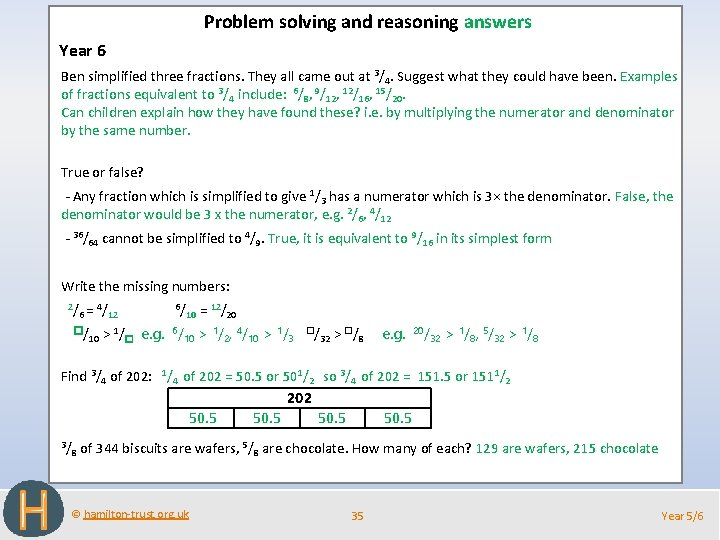Problem solving and reasoning answers Year 6 Ben simplified three fractions. They all came out at 3/4. Suggest what they could have been. Examples of fractions equivalent to 3/4 include: 6/8, 9/12, 12/16, 15/20. Can children explain how they have found these? i. e. by multiplying the numerator and denominator by the same number. True or false? - Any fraction which is simplified to give 1/3 has a numerator which is 3× the denominator. False, the denominator would be 3 x the numerator, e. g. 2/6, 4/12 - 36/64 cannot be simplified to 4/9. True, it is equivalent to 9/16 in its simplest form Write the missing numbers: 2/6 = 4/12 6/10 = 12/20 ☐/10 > 1/☐ e. g. 6/10 > 1/2, 4/10 > 1/3 ☐/ 32 > ☐/ 8 e. g. 20/ 32 > 1/8, 5/32 > 1/8 Find 3/4 of 202: 1/4 of 202 = 50. 5 or 501/2 so 3/4 of 202 = 151. 5 or 1511/2 202 50. 5 3/ 8 of 344 biscuits are wafers, © hamilton-trust. org. uk 50. 5 5/ 50. 5 8 are chocolate. How many of each? 129 are wafers, 215 chocolate 35 Year 5/6